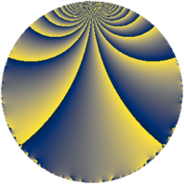Properties

 Label 8280.2.a.beLevel $8280$ Weight $2$ Character orbit 8280.a Self dual yes Analytic conductor $66.116$ Analytic rank $1$ Dimension $2$ CM no Inner twists $1$

Related objects

Newspace parameters

 Level: $$N$$ $$=$$ $$8280 = 2^{3} \cdot 3^{2} \cdot 5 \cdot 23$$ Weight: $$k$$ $$=$$ $$2$$ Character orbit: $$[\chi]$$ $$=$$ 8280.a (trivial)

Newform invariants

 Self dual: yes Analytic conductor: $$66.1161328736$$ Analytic rank: $$1$$ Dimension: $$2$$ Coefficient field: $$\Q(\sqrt{17})$$ Defining polynomial: $$x^{2} - x - 4$$ Coefficient ring: $$\Z[a_1, \ldots, a_{7}]$$ Coefficient ring index: $$1$$ Twist minimal: no (minimal twist has level 2760) Fricke sign: $$1$$ Sato-Tate group: $\mathrm{SU}(2)$

$q$-expansion

Coefficients of the $$q$$-expansion are expressed in terms of $$\beta = \frac{1}{2}(1 + \sqrt{17})$$. We also show the integral $$q$$-expansion of the trace form.

 $$f(q)$$ $$=$$ $$q + q^{5} -\beta q^{7} +O(q^{10})$$ $$q + q^{5} -\beta q^{7} + 2 \beta q^{11} + 2 q^{13} + ( -2 - \beta ) q^{17} + ( -4 + 2 \beta ) q^{19} + q^{23} + q^{25} + ( -6 - \beta ) q^{29} + ( 4 - 3 \beta ) q^{31} -\beta q^{35} + ( -2 + \beta ) q^{37} + ( -6 + \beta ) q^{41} + ( 4 - 4 \beta ) q^{43} -8 q^{47} + ( -3 + \beta ) q^{49} + ( 2 - \beta ) q^{53} + 2 \beta q^{55} + ( -4 - \beta ) q^{59} + ( -2 + 6 \beta ) q^{61} + 2 q^{65} -\beta q^{67} + ( -4 - 3 \beta ) q^{71} + 2 q^{73} + ( -8 - 2 \beta ) q^{77} + 4 \beta q^{79} + ( 8 - 3 \beta ) q^{83} + ( -2 - \beta ) q^{85} + ( -2 + 2 \beta ) q^{89} -2 \beta q^{91} + ( -4 + 2 \beta ) q^{95} -6 q^{97} +O(q^{100})$$ $$\operatorname{Tr}(f)(q)$$ $$=$$ $$2 q + 2 q^{5} - q^{7} + O(q^{10})$$ $$2 q + 2 q^{5} - q^{7} + 2 q^{11} + 4 q^{13} - 5 q^{17} - 6 q^{19} + 2 q^{23} + 2 q^{25} - 13 q^{29} + 5 q^{31} - q^{35} - 3 q^{37} - 11 q^{41} + 4 q^{43} - 16 q^{47} - 5 q^{49} + 3 q^{53} + 2 q^{55} - 9 q^{59} + 2 q^{61} + 4 q^{65} - q^{67} - 11 q^{71} + 4 q^{73} - 18 q^{77} + 4 q^{79} + 13 q^{83} - 5 q^{85} - 2 q^{89} - 2 q^{91} - 6 q^{95} - 12 q^{97} + O(q^{100})$$

Embeddings

For each embedding $$\iota_m$$ of the coefficient field, the values $$\iota_m(a_n)$$ are shown below.

For more information on an embedded modular form you can click on its label.

Label $$\iota_m(\nu)$$ $$a_{2}$$ $$a_{3}$$ $$a_{4}$$ $$a_{5}$$ $$a_{6}$$ $$a_{7}$$ $$a_{8}$$ $$a_{9}$$ $$a_{10}$$
1.1
 2.56155 −1.56155
0 0 0 1.00000 0 −2.56155 0 0 0
1.2 0 0 0 1.00000 0 1.56155 0 0 0
 $$n$$: e.g. 2-40 or 990-1000 Significant digits: Format: Complex embeddings Normalized embeddings Satake parameters Satake angles

Atkin-Lehner signs

$$p$$ Sign
$$2$$ $$-1$$
$$3$$ $$-1$$
$$5$$ $$-1$$
$$23$$ $$-1$$

Inner twists

This newform does not admit any (nontrivial) inner twists.

Twists

By twisting character orbit
Char Parity Ord Mult Type Twist Min Dim
1.a even 1 1 trivial 8280.2.a.be 2
3.b odd 2 1 2760.2.a.p 2
12.b even 2 1 5520.2.a.bh 2

By twisted newform orbit
Twist Min Dim Char Parity Ord Mult Type
2760.2.a.p 2 3.b odd 2 1
5520.2.a.bh 2 12.b even 2 1
8280.2.a.be 2 1.a even 1 1 trivial

Hecke kernels

This newform subspace can be constructed as the intersection of the kernels of the following linear operators acting on $$S_{2}^{\mathrm{new}}(\Gamma_0(8280))$$:

 $$T_{7}^{2} + T_{7} - 4$$ $$T_{11}^{2} - 2 T_{11} - 16$$ $$T_{13} - 2$$ $$T_{17}^{2} + 5 T_{17} + 2$$

Hecke characteristic polynomials

$p$ $F_p(T)$
$2$ $$T^{2}$$
$3$ $$T^{2}$$
$5$ $$( -1 + T )^{2}$$
$7$ $$-4 + T + T^{2}$$
$11$ $$-16 - 2 T + T^{2}$$
$13$ $$( -2 + T )^{2}$$
$17$ $$2 + 5 T + T^{2}$$
$19$ $$-8 + 6 T + T^{2}$$
$23$ $$( -1 + T )^{2}$$
$29$ $$38 + 13 T + T^{2}$$
$31$ $$-32 - 5 T + T^{2}$$
$37$ $$-2 + 3 T + T^{2}$$
$41$ $$26 + 11 T + T^{2}$$
$43$ $$-64 - 4 T + T^{2}$$
$47$ $$( 8 + T )^{2}$$
$53$ $$-2 - 3 T + T^{2}$$
$59$ $$16 + 9 T + T^{2}$$
$61$ $$-152 - 2 T + T^{2}$$
$67$ $$-4 + T + T^{2}$$
$71$ $$-8 + 11 T + T^{2}$$
$73$ $$( -2 + T )^{2}$$
$79$ $$-64 - 4 T + T^{2}$$
$83$ $$4 - 13 T + T^{2}$$
$89$ $$-16 + 2 T + T^{2}$$
$97$ $$( 6 + T )^{2}$$